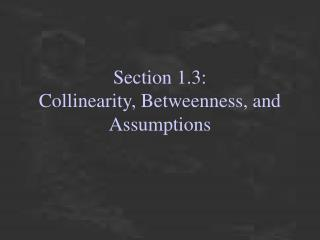# Section 1.3: Collinearity, Betweenness, and Assumptions - PowerPoint PPT PresentationDownload PresentationSection 1.3: Collinearity, Betweenness, and Assumptions

Section 1.3: Collinearity, Betweenness, and AssumptionsDownload Presentation## Section 1.3: Collinearity, Betweenness, and Assumptions

- - - - - - - - - - - - - - - - - - - - - - - - - - - E N D - - - - - - - - - - - - - - - - - - - - - - - - - - -
##### Presentation Transcript

1. Section 1.3:Collinearity, Betweenness, and Assumptions

2. C A B C A B Collinearity • Collinear points are points that lie on the same line • Non-collinear points are points that do not lie on the same line Are A,B, and C collinear? Collinear Noncollinear

3. S R S T R T Betweenness Betweenness occurs when one point is between two others, but… …all three points must be collinear Is S between R and T? Yes No, not collinear

4. Triangle Inequality Any three points can be: • Collinear -One point between others • Noncollinear -Points determine a triangle

5. How to use the Triangle Inequality • If we are given three segment lengths we can determine if they are collinear or make up a triangle. • If 2 measures add up to the third then they make up a line • If the sum of 2 measures is greater than the third then they make up a triangle

6. Example • Determine if the following three segments make up a line or a triangle. • AB=17, BC=24, AC=39 • Add up AB and BC AB+BC=17+24=41 • Compare AB+BC to AC AB+BC>AC • Since the sum of two of the sides is greater than the third side the three sides make up a triangle.

7. What can we assume? Straight lines and angles Collinearity Betweenness Relative positions What can’t we assume? Right angles Congruent angles Congruent segments Relative size of segments or angles Assumptions from diagrams

8. Can assume Cannot assume Example D B E C A

9. Homework time!! p. 20-22 1-14